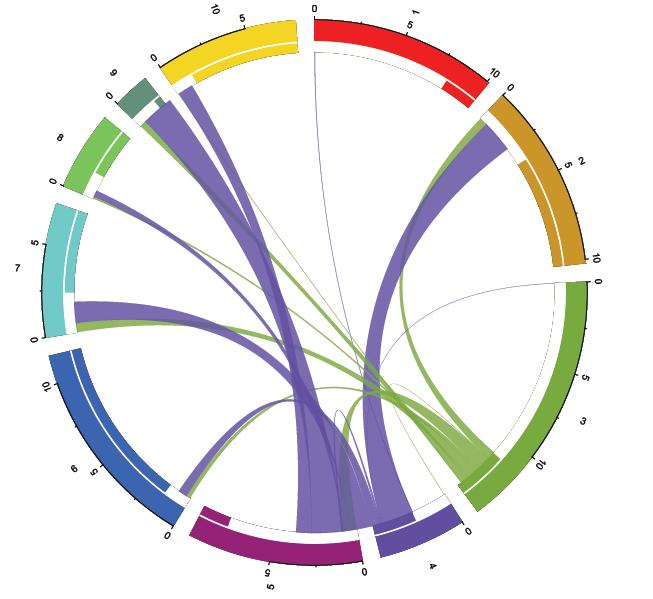# Migration Circos plots in R

We’ve all seen them – colorful, and I daresay, pretty darn informative. Circos plots are fun visualizations of large data-sets. I’ve seen them used in two contexts in comparative genomics – to represent structural variants in homologous chromosome segments in species alignments, and to perhaps represent gene-gene interactions. But there are scores of other interesting applications of these plots to scientific data, a comprehensive list (and yet growing) can be seen here.Circos plot of source-sink migration dynamics between populations “3” and “4” here, with 8 other populations. Width of migration curves indicates amount of migration.

A relatively recent publication by Abel and Sander (2014) in Science on using Circos plots to represent migration prompted me to explore the migest package in R. Scores of studies in molecular ecology and population genetics utilize methods to estimate ancestral or contemporary migration routes between populations, such as MIGRATE-N, IM/IMa2, IMMANC, BayesAss, etc. I am yet to see a migration visualization that comprehensively describes complex migration routes between populations in pop-gen studies. So putting these two together, I thought I’d use migration estimates from one of the above tools, and represent it as a Circos plot. I am going to skip a few steps in creating the data files required to make these plots, but I refer you to some excellent documentation by Guy Abel.

You will need:

File eg. df1.txt – Contains information about the location, RGB colors picked out for each region, and order they should appear in the circle.

```order rgb region
1 255,0,0 1
2 210,150,12 2
3 125,175,0 3
4 117,0,255 4
5 160,0,125 5
6 29,100,255 6
7 0,255,233 7
8 73,255,100 8
9 100,146,125 9
10 255,219,0 10
```

File eg. df2.txt – Contains migration estimates (here I have them scaled to average number of individuals per generation). I used MIGRATE-n for these estimates.

```orig dest m
4 1 36.67
3 2 491.33
4 2 1970
4 3 14
3 4 16.67
3 5 835.33
4 5 106
3 6 252.67
4 6 555.33
3 7 558
4 7 1296.67
3 8 122
4 8 392.67
3 9 391.33
4 9 1967.33
3 10 39.33
4 10 920.67
```

Okay! Now onto reading data, and getting started with plotting.

```library(“migest”)
library("plyr")
names(df1)<-c("order","rgb","region")
```

Now that our files are read, let’s do some housekeeping to the tables.

```df1\$xmin<-0
df1\$xmax<-sum(df2\$m)
n<-nrow(df1)
df1<-cbind(df1, matrix(as.numeric(unlist(strsplit(df1\$rgb,","))),nrow=n,byrow=TRUE))
names(df1)[ncol(df1)-2:0]<-c("r","g","b")
df1\$rcol<-rgb(df1\$r,df1\$g,df1\$b,max=255)
df1\$rcol<-rgb(df1\$r,df1\$g,df1\$b,alpha=200,max=255)
```

We’re now ready to plot! First up – we create the segments around the circle with parameters using the “df1” table. You shouldn’t have to change any of this code.

```library("circlize")
par(mar=rep(0,4))
circos.clear()
track.margin=c(0,0.15),start.degree=90,gap.degree=4)
circos.initialize(factors=df1\$region,
xlim=cbind(df1\$xmin, df1\$xmax))
circos.trackPlotRegion(ylim = c(0, 1),
factors = df1\$region, track.height=0.1,
panel.fun = function(x, y) {
name = get.cell.meta.data("sector.index")
i = get.cell.meta.data("sector.numeric.index")
xlim = get.cell.meta.data("xlim")
ylim = get.cell.meta.data("ylim")
theta = circlize(mean(xlim), 1.3)[1, 1] %% 360
dd <- ifelse(theta < 90 || theta > 270,
"clockwise", "reverse.clockwise")
aa = c(1, 0.5)
if(theta < 90 || theta > 270)  aa =c(0, 0.5)
circos.text(x=mean(xlim), y=1.7,
labels=name, facing = dd, cex=0.6,  adj = aa)
circos.rect(xleft=xlim, ybottom=ylim,
xright=xlim, ytop=ylim,
col = df1\$rcol[i], border=df1\$rcol[i])
circos.rect(xleft=xlim, ybottom=ylim,
xright=xlim-rowSums(m)[i], ytop=ylim+0.3,
col = "white", border = "white")
circos.rect(xleft=xlim, ybottom=0.3,
xright=xlim, ytop=0.32, col = "white",
border = "white")
circos.axis(labels.cex=0.6, direction =
"outside", major.at=seq(from=0,to=floor(df1\$xmax)[i],by=5),
minor.ticks=1,
labels.away.percentage = 0.15)})
```

And we have segments! Now all that’s left to do is plot the migration routes between segments (here populations). Again, you shouldn’t have to change any of this code.

```df1\$sum1 <- colSums(df2\$m)
df1\$sum2 <- numeric(n)
for(k in 1:nrow(df2)){
i<-match(df2\$orig[k],df1\$region)
j<-match(df2\$dest[k],df1\$region)
df1\$sum1[i] + abs(df2\$m[k]/1000)),
sector.index2=df1\$region[j], point2=c(df1\$sum2[j],
df1\$sum2[j] + abs(df2\$m[k]/1000)), col = df1\$lcol[i])
df1\$sum1[i] = df1\$sum1[i] + abs(df2\$m[k]/1000)
df1\$sum2[j] = df1\$sum2[j] + abs(df2\$m[k]/1000)}
```

And behold! I am just in awe of how pretty this plot is – my purple population (4) obviously has a lot more migration than the green one (3). Sort of tells my whole story in one neat figure! As I said before, this is just a bare minimum example. There are plenty of options that can be tweaked to suit your own figure, but it’s still a start. R on!